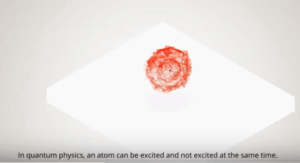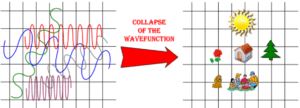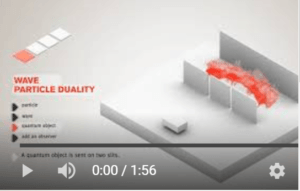Home » Measurement Problem

# Measurement Problem

« Back to Glossary Index

Understanding the Measurement Problem requires understanding the unique role of measurement in quantum mechanics. The entry for measurement describes this role in some detail. The Measurement Problem is, broadly speaking, that we don’t know what triggers a quantum superposition to fall out ofClick for animation of a superposition of an atom in both an excited and a ground state. [Image source: https://toutestquantique.fr/superposition/]the superposition state and choose the particular properties of the quantum particle that we measure. We don’t know the trigger and the mechanism for this transformation. Nor do we know the cause of the selection of one property (such as a particular position) rather than another. Nor do we have a mathematical description of this transformation.The Measurement Problem is the puzzle of the mechanism by which the wavy quantum state (left) abruptly transforms to a particle (right). At least in one sense, this image is metaphorical rather than realistic: the transformation from wave to particle is thought of as occurring at the particle level rather than the macroscopic level of tables and chairs (and picnic blankets). [Image source: David Chalmers and Kelvin McQueen, “Consciousness and the Collapse of the Wave Function” http://consc.net/slides/collapse.pdf]The quantum physicist, Shantena Sabbadini, put it this way:

“But, of course, the [quantum] theory is fundamentally incomplete unless we are able to say what is that something that collapses the wave function. In other words, what is it that makes the macroscopic world, sitting on top of all this weird quantum stuff, appear classical, either-or, well-behaved, solid (kind of), instead of being an entangled mess of head and tail coins and dead and alive cats?”

The mention of “dead and alive cats” is a reference to the Schrodinger Cat Experiment.Click for animation of quantum wave collapsing to a particle. [Image source: https://toutestquantique.fr/superposition/]Physicists give different descriptions of the Measurement Problem. This is, in part, because they take different approaches to assigning physical reality to the equations of quantum physics. They are pretty much in agreement regarding the equations and the experimental results of quantum mechanics. However, those physicists who are concerned about the physical meaning of quantum mechanics subscribe to 20 different interpretations as to what is going on in the physical universe. Some physicists are quite happy to use the calculations of quantum physics to work in technological fields without putting attention on the physical meaning of the calculations.

The original interpretation of quantum mechanics and, to this day, one of the most accepted, is the Copenhagen Interpretation. This is the interpretation most often taught in universities. Within the Copenhagen Interpretation, the Measurement Problem creates riddles with no solutions. The riddles are: 1) What triggers the wave to collapse down to a particle? What is the exact mechanism of collapse? And how can it be described mathematically? 2) What causes the particle to choose a particular property, such as a particular position in a superposition of positions, rather than another? Other interpretations, such as the Transactional Interpretation, solve part of these riddles. And some interpretations, like the De Broglie-Bohmian and Many Worlds, maintain that wave function collapse does not even occur.

In the Copenhagen Interpretation, the Measurement Problem arises from the Schrodinger Equation. When the Schrodinger Equation is solved, the answer is not a number but another equation called the “wave function.” The wave function describes an evolving wave. At no point does the wave function describe transformation of the wave to a particle. Yet, we know, that upon measurement, we will find not a wave, but a particle.

The wave function tells us the probability of where we will detect the particle. Though the wave function omits description of the transformation from wave to particle, it is accurate in predicting the collection of resulting positions in which particles are found in the course of many runs of the experiment.

Mathematically, the moment of measurement is the moment in which the wave function “collapses.” The mathematical description of wave function collapse is that the probability of finding the particle (in this case a photon) where it was detected goes to 100% and the probabilities of finding it in all other positions fall to zero.

This site uses Akismet to reduce spam. Learn how your comment data is processed.